### Geometry Class 8th Mathematics Term 1 Tamilnadu Board Solution

##### Question 1.Choose the correct answer:In the isosceles ∆XYZ, given XY = YZ then which of the following angles are equal?A. ∠X and ∠YB. ∠Y and ∠ZC. ∠Z and ∠XD. ∠X, ∠Y and ∠ZAnswer:Reason: Angles opposite XY and YZ are angle X and Z respectively.[T3]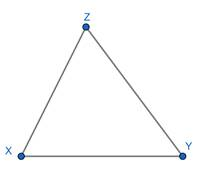Question 2.Choose the correct answer:In Δ ABC and ∆DEF, ∠B = ∠E, AB = DE, BC = EF. The two triangles are congruent under _____ axiomA. SSSB. AAAC. SASD. ASAAnswer:option C.If any two sides and the included angle of a triangle are respectively equal to any two sides and the included angles of another triangle then the two triangles are congruent.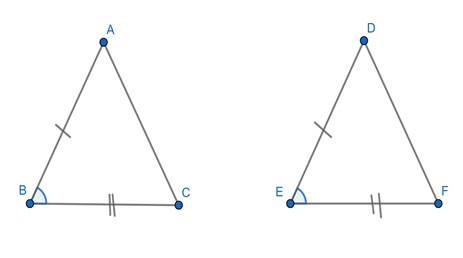Question 3.Choose the correct answer:Two plane figures are said to be congruent if they haveA. the same sizeB. the same shapeC. the same size and the same shapeD. the same size but not same shapeAnswer:Reason: If two geometrical figures are identical in shape and size then they are said to be congruent.Question 4.Choose the correct answer:In a triangle ABC, ∠A = 40o and AB = AC, then ABC is _____ triangle.A. a right angledB. an equilateralC. an isoscelesD. a scaleneAnswer:In a triangle, When two sides are equal then triangle are said to be an isosceles triangle.Question 5.Choose the correct answer:In the triangle ABC, when ∠A = 90o the hypotenuse is ______A. ABB. BCC. CAD. None of theseAnswer:option B[T4]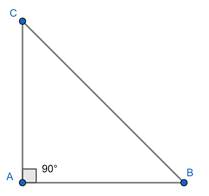Question 6.Choose the correct answer:In the Δ PQR the angle included by the sides PQ and PR isA. ∠PB. ∠QC. ∠RD. None of theseAnswer:[T5]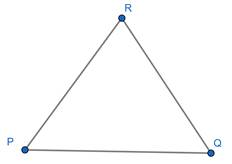Question 7.Choose the correct answer:In the figure, the value of x° is_______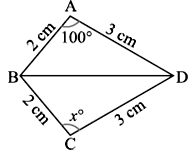A. 80oB. 100oC. 120oD. 200oAnswer:In Δ ABD and Δ CBDAB = CB = 2cmAD = CD = 3cmBD = BD = common∴ Δ ABD ≅ Δ CBD∴ ∠ A = ∠ C⇒ 100° = x°Hence, option B is correct.Question 8.In the figure, ABC is a triangle in which AB = AC. Find x° and y°.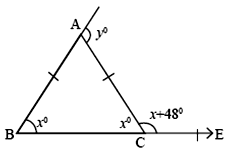Answer:Theorem 2:If a side of a triangle is produced, the exterior angle so formed, is equal to the sum of the two interior angle.∠ ACE + ∠ ACB = 180°⇒ x + 48° + x° = 180°⇒ 2x + 48° = 180°⇒ 2x = 180° - 48°⇒ 2x = 132°⇒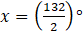⇒ x = 66°Ext. ∠ A = ∠ B + ∠ C⇒ y° = x° + x°⇒ y° = 2x°⇒ y° = 2 × 66°⇒ y° = 132°Question 9.In the figure, Find x°.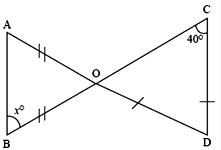Answer:Theorem 1: Sum of all the angles of the triangles is 180°In Δ COBOD = DC∴ ∠ COD = ∠DCO = 40°In Δ AOBOA = OB (Given)∴ ∠ OAB = ∠ OBA = x°∠ OAB + ∠ OBA + ∠ AOB = 180° (Angle Sum Property)⇒ x° + x° + ∠ AOB = 180°⇒ 2x° + ∠ AOB = 180°⇒ ∠ AOB = 180° - 2x°∠ AOB = ∠ COD (opposite angles are equal)180 – 2x° = 40°⇒ 180° = 40 + 2x°⇒ 180° - 40° = 2x°⇒ 140° = 2x°⇒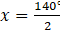⇒ x = 70°Question 10.In the figure ∆PQR and ∆SQR are isosceles triangles. Find x°.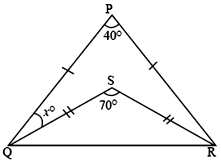Answer:Theorem 1: Sum of all the angles of triangles is 180°Let ∠ PQR be yIn Δ SQR,SQ = SR∴ ∠ SQR = ∠ SRQ∠ SQR + ∠ SRQ + ∠ QSR = 180° (Angle Sum Property)⇒ 2 ∠ SQR + 70° = 180°⇒ 2 ∠ SQR = 180° - 70°⇒ 2 ∠ SQR = 110°⇒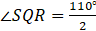⇒ ∠ SQR = 55° = ∠ SRQIn Δ PQR,∠ PQR + ∠ PRQ + ∠ RPQ = 180°⇒ y + y + 40° = 180°⇒ 2y + 40° = 180°⇒ 2y = 180° - 40°⇒ 2y = 140°⇒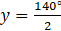⇒ y = 70°∠ PQR = ∠ PQS + ∠ SQR⇒ 70° = x° + 55°⇒ x° = 70° - 55°⇒ x° = 15°Question 11.In the figure, it is given that BR = PC and ∠ACB= ∠QRP and AB ∥ PQ. Prove thatAC = QR.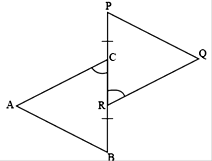Answer:Given: BR = PC and ∠ ACB = ∠ QRP , AB || PQTo Prove: AC = QRProof:In Δ ABC, we haveBC = BR + RCIn Δ PQRPR = PC + RCBut , BR = PC [Given]So, BC = PC + RC and PR = BR + RC⇒ BC = PRSo, in Δ ABC and Δ PQR, we have∠ ACB = ∠ QRP [Given]BC = PR [Proved Above]∠ ABC = ∠ QPR [AB || PQ, alternate interior angles]Thus, Δ ABC ≅ Δ PQR [Angle – Side – Angle]∴ AC = QR [C. P. C. T]Question 12.In the figure, AB = BC = CD, ∠A = xo. Prove that ∠DCF = 3∠A.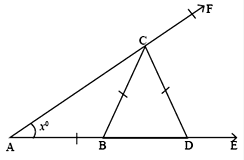Answer:Given: AB = BC = CD and ∠ A = x°To Prove: ∠ DCF = 3 ∠ AProof:In Δ ABCAB = BC [Given]∴ ∠ A = ∠ C = x°Now,∴ ext. ∠ B = ∠ A + ∠ C⇒ Ext. ∠ B = x° + x°⇒ Ext. ∠ B = 2x°In Δ CBDBC = CD [Given]∴ ∠ B = ∠ D = 2x°Now,In Δ ADC,Ext. ∠ DCF = ∠ CDA + ∠ CAD⇒ ∠ DCF = 2x + x⇒ ∠ DCF = 3x⇒ ∠ DCF = 3 ∠ A [ ∠ A = x°, Given]Hence Proved.Question 13.Find x°, y°, z° from the figure, where AB = BD, BC = DC and ∠DAC 30o.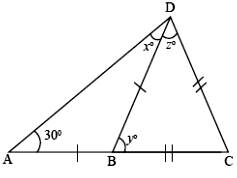Answer:Theorem 1: Sum of all the angles in the triangle is 180°.In Δ ABD,We know that, AB = BD∴ ∠ A = ∠ D⇒ 30° = x°Hence, ∠ A + ∠ B + ∠ C = 180°⇒ 30° + ∠ B + 30° = 180°⇒ ∠ B + 60° = 180°⇒ ∠ B = 180° - 60°⇒ ∠ B = 120°∠ DBA + ∠ DBC = 180° (Sum of adjacent angles is 180°)⇒ 120° + y° = 180°⇒ y° = 180° - 120°⇒ y° = 60°In Δ DBCWe know that, BC = DC∴ ∠ B = ∠ D⇒ y° = z°⇒ 60° = z°Question 14.In the figure, ABCD is a parallelogram. AB is produced to E such that AB = BE. AD produced to F such that AD = DF. Show that ∆FDC ≡ ∆CBE.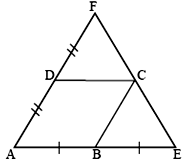Answer:Given: Parallelogram ABCD and AB = BE and AD = FDTo prove: Δ FDC ≡ ΔCBEConstruction: Join DB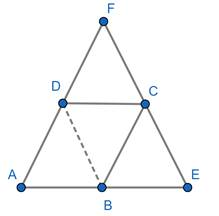Proof:We know that,AB = DC [ opposite sides of parallelogram]BE = DC [AB = BE, because B is the midpoint of AE]Similarly,AD = BC [ opposite sides of parallelogram]DF = BC [ AD = DF, because B is the midpoint of AE]Now, AD||BC and AB∠ A = ∠ B [corresponding angles] …(1)Now, AB||CD and AD∠ A = ∠ D [corresponding angles] …(2)∴ ∠ B = ∠ D (From 1 and 2)In Δ FDC and Δ CBEFD = CB [Proved Above]DC = BE [Proved Above]∠ D = ∠ B [Proved Above]Thus, Δ FDC ≡ Δ CBEHence Proved.Question 15.In figure, BO bisects ∠ABC of ∆ABC. P is any point on BO. Prove that the perpendicular drawn from P to BA and BC are equal.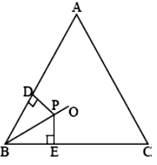Answer:Given: A Δ ABC in which BO is bisector of ∠ABCAlso, we have PD ⊥ AB and PE ⊥ BCTo Prove: PD = PEProof:In Δ PBD and Δ PBEPB = PB [common]∠ PBD = ∠ PBE [ given]∠ PDB = ∠ PEB = 90° [Given]Thus, Δ PBD ≅ Δ PBE [Angle – Angle – Side]∴ PD = PEHence Proved.Question 16.The Indian Navy flights fly in a formation that can be viewed as two triangles with common side. Prove that ∆SRT ≡ ∆QRT, if T is the midpoint of SQ and SR = RQ.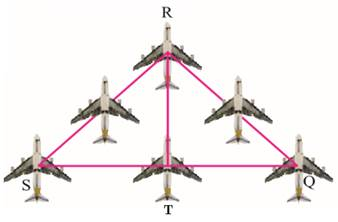Answer:Given: T is the mid-point of SQ and SR = RQTo Prove: Δ SRT ≅ Δ QRTProofIn Δ SRT and QRTRT = RT [common]ST = QT [T is the mid-point of SQ]SR = RQ [Given]Thus, Δ SRT ≅ Δ QRT [Side – Side – Side]Hence Proved.

PDF FILE TO YOUR EMAIL IMMEDIATELY PURCHASE NOTES & PAPER SOLUTION. @ Rs. 50/- each (GST extra)

HINDI ENTIRE PAPER SOLUTION

MARATHI PAPER SOLUTION

SSC MATHS I PAPER SOLUTION

SSC MATHS II PAPER SOLUTION

SSC SCIENCE I PAPER SOLUTION

SSC SCIENCE II PAPER SOLUTION

SSC ENGLISH PAPER SOLUTION

SSC & HSC ENGLISH WRITING SKILL

HSC ACCOUNTS NOTES

HSC OCM NOTES

HSC ECONOMICS NOTES

HSC SECRETARIAL PRACTICE NOTES

# 2019 Board Paper Solution

HSC ENGLISH SET A 2019 21st February, 2019

HSC ENGLISH SET B 2019 21st February, 2019

HSC ENGLISH SET C 2019 21st February, 2019

HSC ENGLISH SET D 2019 21st February, 2019

SECRETARIAL PRACTICE (S.P) 2019 25th February, 2019

HSC XII PHYSICS 2019 25th February, 2019

CHEMISTRY XII HSC SOLUTION 27th, February, 2019

OCM PAPER SOLUTION 2019 27th, February, 2019

HSC MATHS PAPER SOLUTION COMMERCE, 2nd March, 2019

HSC MATHS PAPER SOLUTION SCIENCE 2nd, March, 2019

SSC ENGLISH STD 10 5TH MARCH, 2019.

HSC XII ACCOUNTS 2019 6th March, 2019

HSC XII BIOLOGY 2019 6TH March, 2019

HSC XII ECONOMICS 9Th March 2019

SSC Maths I March 2019 Solution 10th Standard11th, March, 2019

SSC MATHS II MARCH 2019 SOLUTION 10TH STD.13th March, 2019

SSC SCIENCE I MARCH 2019 SOLUTION 10TH STD. 15th March, 2019.

SSC SCIENCE II MARCH 2019 SOLUTION 10TH STD. 18th March, 2019.

SSC SOCIAL SCIENCE I MARCH 2019 SOLUTION20th March, 2019

SSC SOCIAL SCIENCE II MARCH 2019 SOLUTION, 22nd March, 2019

XII CBSE - BOARD - MARCH - 2019 ENGLISH - QP + SOLUTIONS, 2nd March, 2019

# HSCMaharashtraBoardPapers2020

(Std 12th English Medium)

HSC ECONOMICS MARCH 2020

HSC OCM MARCH 2020

HSC ACCOUNTS MARCH 2020

HSC S.P. MARCH 2020

HSC ENGLISH MARCH 2020

HSC HINDI MARCH 2020

HSC MARATHI MARCH 2020

HSC MATHS MARCH 2020

# SSCMaharashtraBoardPapers2020

(Std 10th English Medium)

English MARCH 2020

HindI MARCH 2020

Hindi (Composite) MARCH 2020

Marathi MARCH 2020

Mathematics (Paper 1) MARCH 2020

Mathematics (Paper 2) MARCH 2020

Sanskrit MARCH 2020

Sanskrit (Composite) MARCH 2020

Science (Paper 1) MARCH 2020

Science (Paper 2)

Geography Model Set 1 2020-2021

MUST REMEMBER THINGS on the day of Exam

Are you prepared? for English Grammar in Board Exam.

Paper Presentation In Board Exam

How to Score Good Marks in SSC Board Exams

Tips To Score More Than 90% Marks In 12th Board Exam

How to write English exams?

How to prepare for board exam when less time is left

How to memorise what you learn for board exam

No. 1 Simple Hack, you can try out, in preparing for Board Exam

How to Study for CBSE Class 10 Board Exams Subject Wise Tips?

JEE Main 2020 Registration Process – Exam Pattern & Important Dates

NEET UG 2020 Registration Process Exam Pattern & Important Dates

How can One Prepare for two Competitive Exams at the same time?

8 Proven Tips to Handle Anxiety before Exams!